# Terminology used in Addition Subtraction Multiply Division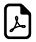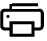## Addition Subtraction Multiplication Division (Terms Used)

Operations Vocabulary Explanation Example
Addition Augend Number to which another is added.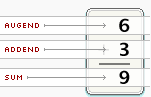Addend Number which is added to another.
Sum Sum is the result of an addition

Subtraction Minuend The first number in a subtraction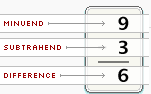Subtrahend Number that is to be subtracted. 2nd no.in a subtraction
Difference Difference is the result of a subtraction problem.

Multiplication Multiplicand Number which is multiplied by another numberMultiplier Number with which multiply is called the multiplier
Product Product is the result of multiplying.

Division Dividend The number being divided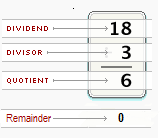Divisor Number that will divide the dividend exactly
Quotient The Result of Division
Remainder Number "left over" after dividing one Number by another

3 + 2 = 5 ,

Here, 3 is Augend

5 is Sum

#### Subtraction Example:

23 - 2 = 21 ,

Here, 23 is Minuend

2 is Subtrahend

21 is Difference

##### Multiplication Example:

5 x 1 = 5 ,

Here, 5 is Multiplicand

1 is Multiplier

5 is Product

###### Division Example:

10 ÷ 2 = 5 ,

Here, 10 is Dividend

2 is Divisor

5 is Quotient

0 is Remainder ( No left over after dividing)

###### These vocabulary of addition,subtraction,multiply and division is the basic things in general mathematics###See Also ►

Table of Squares, Cubes, Perfect Fourths & Fifths